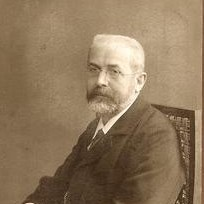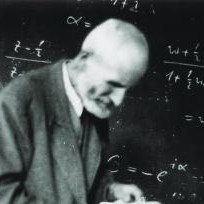# The Perron Frobenius Theorem

 A nxn matrix with positive entries has a unique largest eigenvalue.

A vector is stochastic, if it has non-negative entries which add up to 1. A stochastic matrix is a matrix for which the column vectors are stochastic vectors We have seen in a homework that the product of stochastic matrices is stochastic. It follows that such a stochastic matrix has a determinant which is in absolute value smaller than 1. It also follows that if a stochastic matrix A has the property that An has only positive entries, then A has a unique largest eigenvalue.## Oskar Perron

Oskar Perron (1880 - 1975) did great research until old age.

## Ferdinand Frobenius

Ferdinand Georg Frobenius (1849-1917) worked for 17 years at the ETH in Zürich.

 Ethan Addicott made a nice observation that if take a stochastic matrix and invert it then the inverse has again the property that the sum of the column vectors add up to 1. He saw that row reducing [A | 1] leads to [1 A-1]. The sum in each column can be remain constant during row reduction. It follows that also the inverse matrix has this property. But the column vectors of the inverse are no more stochastic in general. [Added, March 13, 2021: Christian Stahn pointed out that the argument is easier to see without restricting row reduction types by observing that the vector [ 1 1 ... 1 | -1 -1 .. -1 ]T remains in the null space when row reducing [ A | I ] to [ I | A-1 ]. ] The inverse of a stochastic matrix with positive entries is not stochastic because the determinant of the inverse is then larger than one. Can the inverse of a stochastic matrix be stochastic? Yes, if the matrix is doubly stochastic and orthogonal. In that case, the inverse is the transpose and still stochastic.